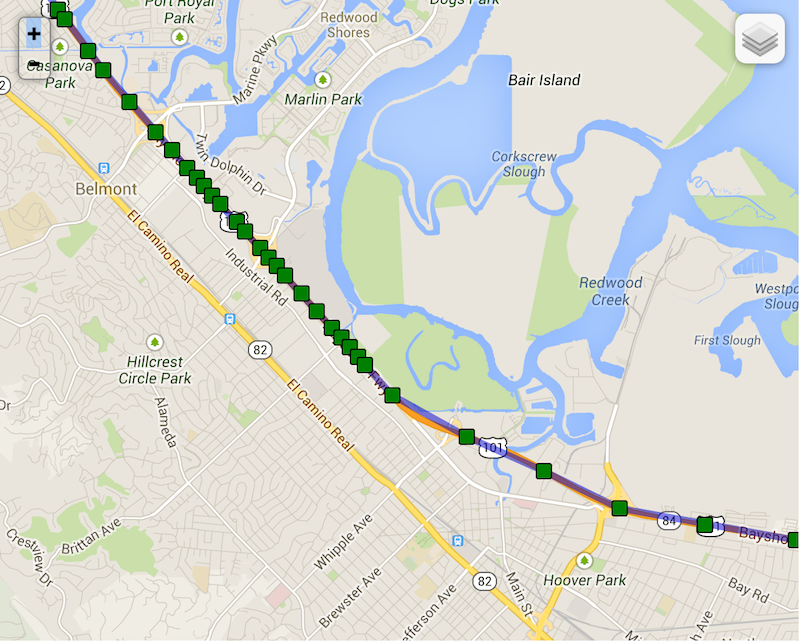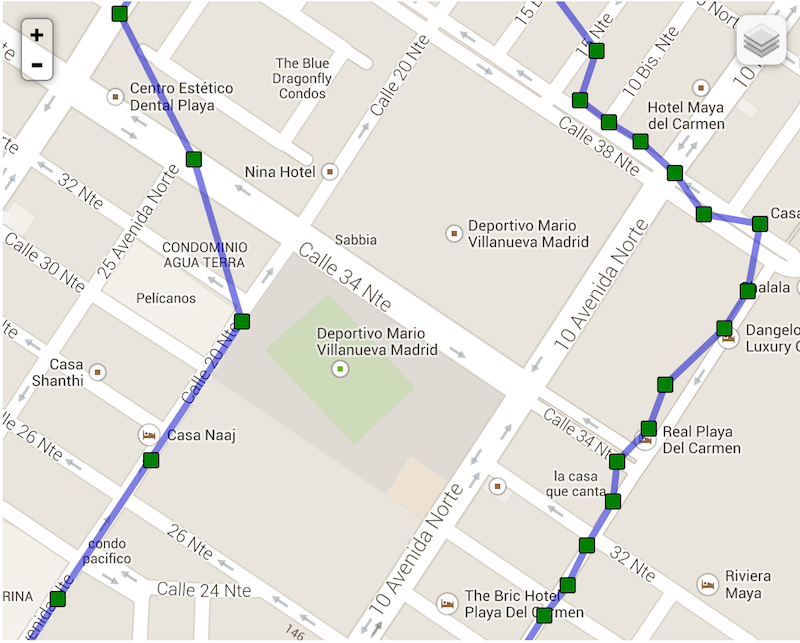# distanceFilter property Null safety

double? distanceFilter

The minimum distance (measured in meters) a device must move horizontally before an update event is generated.

However, by default, `distanceFilter` is elastically auto-calculated by the plugin: When speed increases, `distanceFilter` increases; when speed decreases, so too does `distanceFilter`.

NOTE:

`distanceFilter` is auto-scaled by rounding speed to the nearest `5 m/s` and adding `distanceFilter` meters for each `5 m/s` increment.

For example, at biking speed of 7.7 m/s with a configured `distanceFilter: 30`:

``````  rounded_speed = round(7.7, 5)
=> 10
multiplier = rounded_speed / 5
=> 10 / 5 = 2
=> 2 * 30 = 60 meters
``````

At highway speed of `27 m/s` with a configured `distanceFilter: 50`:

``````  rounded_speed = round(27, 5)
=> 30
multiplier = rounded_speed / 5
=> 30 / 5 = 6
adjusted_distance_filter = multiplier * distanceFilter * elasticityMultipiler
=> 6 * 50 = 300 meters
``````

Note the following real example of background-geolocation on highway 101 towards San Francisco as the driver slows down as he runs into slower traffic (locations become compressed as distanceFilter decreases)Compare now background-geolocation in the scope of a city. In this image, the left-hand track is from a cab-ride, while the right-hand track is walking speed.## Implementation

``````double? distanceFilter;

``````